Email Us: info@lupinepublishers.comCall Us: +1 (914) 407-6109   57 West 57th Street, 3rd floor, New York - NY 10019, USAISSN: 2637-4609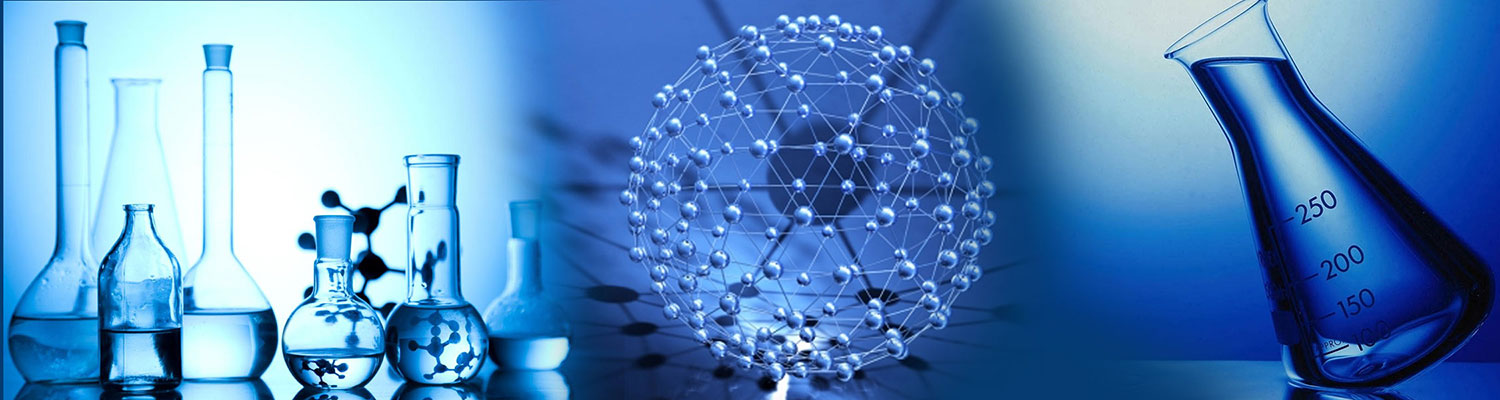Synthesis and Characterization of Poly (1,4-Benzenedimethylene Phthalate) and the Study of its ability to sorb Pb(II), Cd(II), and Zn(II) ions Volume 1 - Issue 1

Aisha N Al-Blawi, Fawwaz I Khalili* and Bassam A Sweileh

• Department of Chemistry, The University of Jordan, Jordan

Received: January 11, 2018;   Published: January 23, 2018

Corresponding author: Fawwaz I Khalili, Faculty of Science, Department of Chemistry, The University of Jordan, Amman, Jordan 11942, Jordan

DOI: 10.32474/AOICS.2018.01.000101

Abstract

Poly (1,4-benzenedimethylene phthalate) was synthesized by condensation of phthaloyl chloride with 1,4-benzenedimethanol in the presence of pyridine in dry THF at 30 °C . The resulting polymer white powder was characterized by viscosity measurement, FT-IR, 1H and 13C NMR, elemental analysis, thermal (TGA-DSC) methods and scanning electron microscopy. The uptake properties of Pb(II), Zn(II) and Cd(II) metals by the polymer from aqueous solutions were studied by the batch and column techniques as a function of pH, temperature, concentration and contact time. The uptake increased with increasing pH reaching a maximum at pH = 6.00 for all ions. The polymer showed high uptake capacities toward Pb(II) and Zn(II) ions, but medium uptake capacity toward Cd (II). The linearized forms of Langmuir, Freundlich and Dubinin-Radushkevich adsorption isotherms indicate studied ions Pb(II), Zn(II), and Cd(II). The adsorption capacity follow the order: Pb(II) > Zn(II) > Cd(II).

The thermodynamic functions, ΔGǂ, ΔHǂ and ΔS° were determined for Pb(II), Zn(II) and Cd(II); the values of AG° indicated that the adsorption process of these metal ions on the polymer is favorable. In this work, the values of ΔGǂ, ΔGǂ and ΔSǂ were determined by using Eyring-Polanyi equation and Arrhenius equation. The results indicated that ΔGǂ=72.8, 78.6, 87.8kJ/mol for Pb, Zn, Cd ions, respectively. The column experiments for metal ion uptake were conducted at pH= 6.0, 25.0 °C, and initial concentration of 150.0mg/L. The loaded concentrations were 76.42, 49,05 and 28.47 ppm for Pb, Zn, Cd ions, respectively. The efficiency for recovery of metal ions after adsorption by treatment of the loaded polymer with 0.1M HNO3, 0.1M EDTA, gave good percent recovery for 0.1M HNO3

Keywords: Benzendimethanol; Phthalate polymers; Condensation polymerization; Polymers uptake; Metal ion uptake; Adsorption

Introduction

Heavy metals are known for their toxicity to living organisms. Their concentrations in the environment increase with the increase of industrial activities. Most important, these metals are not biodegradable and tend to accumulate in living organisms, and causes serious health problems . Allot of methods were reported for removal of metal ions from aqueous solutions; these include filtration, adsorption, chemical precipitation and ion exchange . Different approach for removal of metal ions from water streams which have been increased in recent years , is the adsorption of metals by polymers. This approach is characterized by high effectiveness, high adsorption capacity, and high selectivity, fast kinetics of metal-ion uptake, high mechanical strength, chemical resistance and recyclability of the polymer [4,5].

According to their methods of preparation, synthetic polymers can be divided into three major types:

A. Chain Reaction (Addition) Polymers: These polymers are formed by addition to the double bond of monomers containing carbon-carbon double bonds, called vinyl monomers, without the loss of small molecules. The repeating units of addition polymers have the same composition as the monomer .

B. Condensation Polymers: these polymers are formed by condensation reaction of monomers containing organic functional groups. These reactions usually involve elimination of small molecules (e.g., water, methanol, and ethanol) .

C. Other Polymers May Be Prepared by Modification of Polymers by: I- Attachment of an appropriate specific ligand groups to the polymer chains. This method has been preferred since functional groups containing ligands can easily be attached to polymers than inorganic supports. [7,8]f The attachment of dithiocarbamates to polystyrene cross linked with 2% divinylbenzene is an example of this type . II- Polymerization of monomers to which ligand groups are attached. Polymers with ester linkages in their main chain are used in many applications such as biomedical matrices, liquid crystals, fibers, and heat resistant materials . Polyesters are characterized by higher adsorption capacities, efficiencies as well as high selectivity to some metal ions.  Synthetic polyesters may be prepared by polycondensation of diacids with diols, diaciddichlorides with diols and ester interchange reaction of diesters with diols.

There are two types of polyesters: Aliphatic polyesters and aromatic polyesters. The synthesis of aliphatic polyesters has been well established for several years. However, these polyesters possess low thermal stability due to their low melting points and glass transition temperatures owing to their low molecular weight. These properties resulted in limited usage and few applications of aliphatic polyesters, yet they showed potential as biodegradable polymers. On the other hand, aromatic polyesters display an excellent pattern of physical properties. They are strongly resistant to hydrolysis, bacterial and fungal attack, they also remain unaltered in the environment [11,12], Combining aromatic and aliphatic units in the same polyester chain has been envisaged as an attractive approach to obtain novel products encompassing biodegradability and high performance properties .

There are a lot of polymers were prepared and their sorption behavior was studied in our laboratory,such aspoly (1,4-cyclohexanedimethylene oxalate), Poly (bisphenol A oxalate), poly-cis,trans (1,3-cyclohexylene oxalate), poly (bisphenol- Aphthalate), poly (bisphenol-Acis-1,2 cyclohexanedicarboxylate), poly (1,4-yclohexanedimethanolphthalate) and poly (1,4-cyclohexanedimethanol succinate).

Experiment

Materials

The chemicals were obtained from commercial sources as either analytical reagent grade or chemically pure grade and were used as received. The chemicals were purchased from the corresponding companies: Phthaloyl dichloride, 1,4-benzenedimethanol, cadmium(II) nitrate tetrahydrate and zinc(II) nitratehexahydrate from BDH; N,N-4-dimethylaminopyridine (4- DMAP), dimethylsulfoxide (DMSO) from Acros, pyridine (GPR), nitric acid (65%), hydrochloric acid (36.5 %), and disodium ethylenediaminetetraacetate(EDTA)(Scharlau), sodiumperchlorate (SIGMA), tetrahydofuran (THF) (GCC) and lead(II) nitrate (PRS Paureac) and 4-dimethylaminopyridine (4-DMAP)from Acros.

Preparation of The Polymer

Poly(1,4-benzenedimethylenephthalate) was synthesized by polycondensation using single phase organic solvent polymerization. 1,4-benzenedimethanol ( 4.97g, 0.036 mol), pyridine (8.54g, 0.108mol) and a catalytic amount of 4-DMAP were dissolved in THF (60 mL). To this solution, a solution of phthaloyl chloride (7.307 g, 0.036mol) in THF (30mL) was added drop wise with stirring. The reaction mixture was stirred for 1 h at (30-35) oC and then for 3 days at room temperature. The polymer precipitated as a white solid from the THF solution. The solvent was evaporated and the solid was dissolved in chloroform (150mL) and washed with water (2x500mL), (6% v/v) HCl solution (1 x 150mL), and finally with distilled water (3x500mL). The chloroform solution was dried over anhydrous sodium sulfate, and was then concentrated to about 100 mL of solution. The polymer was precipitated by drop wise addition of chloroform solution to 500.0mL of methanol. The precipitated polymer was then filtered and dried at 55.0 oC under vacuum to give a white powder 66.0g, (68 % yield).

Preparation of Stock Solutions

Stock solutions (1000.0 mg/L) of the three metal ions were prepared by dissolving specific amounts of the salts of Pb(II), Zn(II), and Cd(II), in 0.1 M NaClO4 which was adjusted to the desired pH. The stock solutions were used to prepare solution with different concentrations (20.0, 40.0, 50.0, 60.0, 80.0, 100 and 150.0mg/L). The dilution is achieved by using 0.10 M NaClO4 and adjusted by 0.10 M HClO4 to achieve the desired pH= 4.00, 5.00 and 6.00.

Study of Metal Uptake Characteristics of The Polymers By Batch Technique

The metal uptake characteristics for each metal ion were studied using batch equilibrium technique. An aqueous solution of known metal ion concentration (25.0mL) was added to polymer powder (0.10 g), the mixed solutions were mechanically shaken, after a certain period of time at 25.0 oC, 35.0 oC, 45.0 oC, the mixture was filtered and the amount of the metal ion remaining in the filtrate solution was determined by atomic absorption spectrometry after constructing up an analytical calibration curve for each element (Pb(II), Zn(II), and Cd(II)).

The Rate of Metal Ion Uptake

Experiments for determining the equilibrium time for the metal ion uptake process involving 100.0mg ± 0.1 mg of the polymer was swelled with 25.0mL of metal ion solution containing 150mg/L metal ion at different pH, and the solution was mechanically shaken. The contact time was varied from 5 minutes to 48 hours at 25.0 oC, 35 oC, 45 oC. The mixture was filtered and the amount of the metal ion remaining was determined with atomic absorption spectrometry. The amount of metal ion uptake by the polymer (qe), may be obtained from the following relation: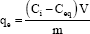qe: Metal ion uptake by the polymer (in mg M(II)/ g polymer).

Ci: Initial metal ion concentration (mg/L).

Ceq : The residual concentration of the metal ion in solution at equilibrium (in mg/L).

V: volume of solution (L).

m: mass of polymer (g).

And the percentage of metal ion loading by the polymer expressed as % uptake was :

Effect of pH on The Metal-Ion Uptake

Similar experiments were also carried out, under different pH values of 4.00, 5.00 and 6.00 for fixed contact time of 24 hours to determine the effect of pH on the metal ion uptake by the polymer.

The adsorption of Pb(II), Zn(II), and Cd(II) was carried out by taking a known mass of 100.0g ± 0.1mg of the polymer swelled with 25.0mL of solutions of concentration variation ranging from (20.0- 150.0)mg/L for each metal, under different pH values of 4.00, 5.00 and 6.00 and different temperatures (25.0, 35.0 and 45.0) oC.

Metal Ion-Uptake By The Polymer Using Column Experiment

Glass column of 30.0 cm length and 1.5cm inner diameter was used in this experiment. The column was packed with 1.00g ± 0.1mg dried polymer. A sample volume of 150.0mL containing Pb(II) of 1000mg/L was passed through the column at a flow rate of 1.0mL/4min. The eluate was collected in a 100.0mL volumetric flask, and concentration of the metal ion was then determined by AAS. The same experimental conditions were used for the determination of Zn(II), and Cd(II) ions uptake, where the sample which passed through the column was 150mg/L of these metal ions.

Desorption studies

The desorption of the Pb(II), Zn(II), and Cd(II), ions was carried under column condition, where the polymer was loaded with each metal ion as described before, using 50.0mL of two eluting agents, 0.10M HNO3 and 0.10M EDTA were used for polymer recovery from adsorbed metal ion, keeping the flow rate of elution at (1mL/4min). The concentration of metal ion in the eluate was collected in five 10.0mL portions, and was then determined by AAS.Water regain (α):

Water regain is defined as the amount of water absorbed by 1000.0mg ± 0.1 mg of polymer . A sample of dry polymer was suspended in water, and was left for 2 and 24 hours. The polymer was filtered and weighed, dried at 60.0 oC and then re-weighed. Water regain (α) was calculated from the mass difference (eq).Results And Discussion

Polymer synthesis

The synthesis of poly (1,4-benzenedimethylene phthalate), from equimolar amounts of 1,4-benzenedimethanol and phthaloyl chloride was performed by solution polycondensation in THF at 30.0 °C in the presence of excess pyridine as acid scavenger and 4-DMAP as the catalyst. The reaction proceeded by pyridine- catalyzed nucleophilic displacement of the chloride of the phthaloyl chloride with the alcoholic group of 1,4-benzenedimethanol. The relatively high yield of the polymer may have been due to the high reactivity of phthaloyl chloride group. The resulted polymer, which was obtained as a powder was found to be insoluble in many common organic solvents such as tetrahydrofuran (THF), diethyl ether, acetone and methanol but soluble in chloroform.

Solution viscosity

The inherent viscosity of poly(1,4-benzenedimethylene phthalate) solution was calculated from viscosity measurements of dilute polymer solutions (0.5g/dL) in chloroform at 25 °C. The polymer had an inherent viscosity of 0.22dL/g. This value indicates that the polymer had an intermediate inherent viscosity which implies that it had moderate molecular masses. This value is higher than the those obtained at 0-5° for poly(bisphenol-Aphthalate), poly(bisphenol-Asuccinate), and poly(cyclohexanedimethylene phthalate) which had the values of 0.11dL/g , 0.13dL/g, and 0.11dL/g, respectively .

Infrared spectroscopy

The polymer was analyzed by FT-IR spectroscopy. The FT-IR spectrum exhibits characteristic absorption bands for the major bonds involved in the polymer. The FTIR spectrum (Figure 1) showed two strong absorption bands for the stretching vibration of the carbonyl group (C=O) of the phthalate ester group at 1726cm-1 and for C-O-C in the range from 1124 to 1279cm-1. Another strong IR band was observed at 2951cm-1 assigned to the C-H stretching in the 1,4-benzendimethanol moiety. These wave numbers, which are typical for the ester group are conformed to the reported literature , and thus confirm the formation of the postulated polymer Scheme 1 and Figure 1.

Scheme 1: Preparation of poly (1,4-benzenedimethylene phthalate) polymer.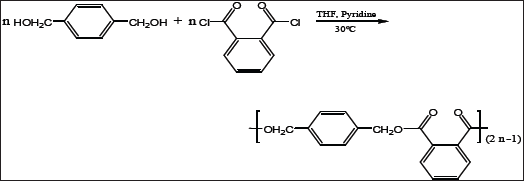Figure 1: IR spectrum of poly(1,4-benzendimethayl phthalate) polymer.The Nuclear magnetic resonance (nmr) spectra for polyesters

Figure 2: 1H-NMR spectrum of the polymer.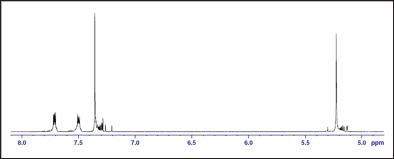1H-NMR spectrum: The polymer was analyzed by NMR spectroscopy in order to elucidate its chemical structure and support its formation. In the 1H-NMR spectrum of poly(1,4- benzendimethylene phthalate), the signal of the aromatic protons of the phenylene ring of benzendimethylene unit was observed as singlet at δ = 7.36ppm, whereas that of the aliphatic methylene protons attached to the oxygen of the ester group was observed as singlet at δ = 5.22ppm. The aromatic methylene protons of the phthalate unit in ortho and meta positions to the ester were observed at δ = 7.71ppm and 7.50ppm, respectively. The 1H-NMR spectrum is shown in Figure 2. In the 13C-NMR spectrum of poly(1,4- benzendimethylene phthalate), the signal of the aromatic carbon atoms of the phenylene ring of benzendimethylene unit appeared at δ = 129ppm. The signal of the quaternary aromatic carbon atoms appeared at δ = 136ppm. The signal of the aliphatic methylene carbon atoms attached to the oxygen of the ester group appeared at δ = 67ppm. The signal of the quaternary aromatic carbon atoms of the phthalate unit to which the ester group is attached appeared at δ = 132ppm. The signals of the aromatic carbon atoms in ortho and meta positions to the ester group appeared at δ = 129ppm and 133ppm, respectively. The signal of the carbonyl carbon atom of the ester group appeared at δ = 167 ppm. The 13C-NMR spectrum of the polymer is shown in Figure (2), and the1H-NMR and 13C-NMR spectral data for the polymer assigned to the various proton and carbon atoms are presented in Table 1 (Figures 3 & 4).

Figure 3: 13C-NMR spectrum of the polymer.Figure 4: DSC thermo gram of polymer.Table 1: Thermal stability of polymer.Thermal Properties

The thermal properties of polyesters synthesized were also investigated by differential scanning calorimetry (DSC), and thermogravimetric analysis (TGA) under dry N2 atmosphere.

Thermal Transition

The thermal properties of polymer were investigated with DSC and TGA. The Tg value of the polymer was 52°C. This value is considered lower than expected for such an aromatic polymer such as poly(ether carbonate) containing aromatic -aromatic ether showed Tg values from room temperature up to 47°C  and polyquinoxalines and other aromatic polymers were studied Tg from 215.5 to 394.5°C . This Tg value may have been due to the imparted flexibility effects of the aliphatic methylene groups of the 1,4 -benzenedimethanol. The presence of the aliphatic moieties in the polymer backbone imparted flexibility to polymer segments to move under the effect of temperature. This ease of motion is reflected in the slightly low Tg value of the polymer . The DSC thermogram of the polymer is shown in Figure 4.

Thermal stability

The thermal stability of the polymer was investigated by TGA under dry nitrogen. Table 1 summarizes the initial thermal decomposition (onset) temperature Tid, T5%d , T10%d , and T50%d decomposition temperatures, which correspond to the temperatures at which 1, 5, 10, and 50% loss of mass of polymer occurred, respectively. The table 2 also shows the residual mass percent remaining after heating the polymer to 673.1 °C which was found to be 8.74%. The thermogram of the polymer Figure 5 displayed a typical one-stage characteristic with a relatively fast mass loss occurring at temperatures between 400 and 450 °C. The fast mass losses may have been due to decomposition of the polymer backbone. These values are higher than the corresponding values obtained in the case of poly (1,4-cyclohexandimethlene- phthalate), which occurred between 350-450°C and the residual mass equal 0.44% .

Table 2: Several reported metal uptake values by chelating polymers in the literature.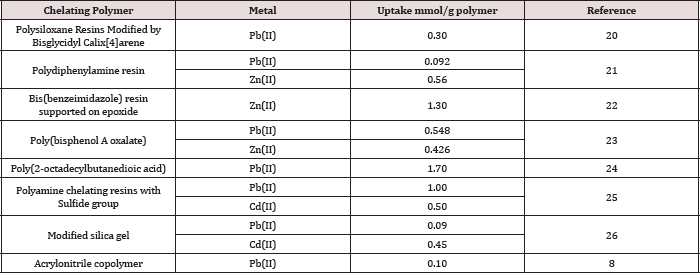Figure 5: TGA thermogram of poly(1,4-benzenedimethayl phthalate) polymer.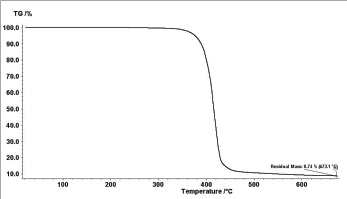Morphological Characterization

The surface ofthe polymer was also characterized by SEM before and after metal sorption. The SEM micrographs for the polymer synthesized are shown in Figure 6. The pores are distributed on the rough surface of the polymer, the small pores which are similar to the forms of flowers are located on the surface of the rods with an average size <5|im. The existence ofthese pores provides convenient diffusion channels for metal ions into the interior of the polymer when it is used for adsorption of metal ions from aqueous solution. The interior structure of the polymer showed randomly distributed large gaps and air pockets created during polymerization. The spongy structure of the inner rods maximize the contact surface between the polymer and the solution which led to increased metal ion uptake, this is shown in Figure 6a for the polymer before metal sorption. However, after adsorption of metal ion by the polymer, a slight loss occurred to the composition of surface features and of the channel and a small part of its surface became smooth. This is represented for the polymer surface loaded with Cd(II) ions and is shown in Figure 6b & c. It has been found by SEM investigation that loading the polymer with zinc ions changed its surface topography, increased the proportion of smooth surface and resulted in narrow channels and pores Figure 6d & 6e. The highest uptake of metal ions by polymer was for Pb(II), the polymer loaded with lead ions was studied by SEM to observe the changes in surface topography that took place, the entire surface became smooth and the pores disappeared due to full metal ion coverage. SEM for our polymer was similar to that of poly(cychlohexandimethelen succinate) (Al- Dweri, 2010). The images are presented in Figure 6f-6i.

Figure 6: SEM images of poly(1,4-benzenedimethelen phthalate).Water Regain or Water Content ( α )

Water regain experiment was performed to determine the water regain ratio (α) for the polymer. Water regain is usually correlated with the hydrophilic character of the polymer, the higher the water regains, and the more hydrophilic the polymer is. The water regain value for the polymer was found to be 0.019 g/g after 2h of stirring and 0.029g/g after 24h stirring, this indicates that the polymer has a low hydrophilic character. These values are higher than the corresponding values obtained by poly(1,3-cyclohexylene oxalate) polymer which was found to have values of 0.011g/g and 0.014g/g, respectively. Based on these values the polymer is considered to be low hydrophilic in nature  and these values are smaller than the corresponding values obtained for poly (1,4-cyclohexanedimethylene oxalate) polymer which was found to have values of 0.064g/g and 0.087g/g . Principally, the water molecules are polar and would interact with the polar groups of the polymer (the ester group), this interaction explains the water regain properties of the polymer.

The rate of metal ion uptake by the Polymer

The adsorption kinetics of metal ions on the surface of the polymer was investigated as shown in Figure 7 for example. The adsorption of metal ions increase with time until complete saturation.

Figure 7: Metal uptake as a function of contact time, at pH = 6, T = 45 °C, and initial concentration of 150ppm.Using linearized Langmuir and linearized Freundlich isotherm as analyzed to determine adsorption isotherms for metal ion Pb(II), Zn(II) and Cd(II) at different pH values (4.00, 5.00 and 6.00) and different temperatures (25.0 oC, 35.0 oC and 45.0 oC) in the range of concentrations from 20.0 to 150.0mg/L. The adsorption isotherms results are presented in Table 3 and shown in Figure 8 & (Table 2) (20-23).

Figure 8: Plots of a) adsorption isotherm b) Linearized Freundlich c) Linearized Langmuir of Pb(II) at pH = 6 and 45 °C.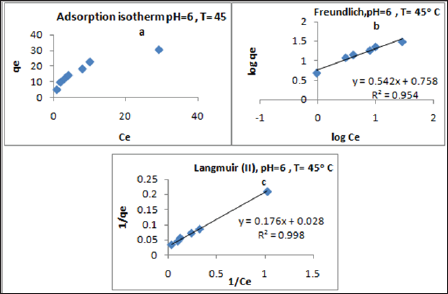Table 3: R2, qm, and KL values obtained from Langmuir plotsIn the Langmuir model, the values of correlation regression coefficient (R2) are greater than 0.90 and had excellent linearity. This indicates that homogenous sites of interaction are better to describe the process and the maximum sorption capacities (qm) deduced from these results indicated that the polymers has the highest capacity towards Pb(II) ions, but it shows a lower capacity towards Cd(II). It is observed that the adsorption capacity (qm) increases as the temperature and pH values increase for all investigated metal ions. Comparing the values of qm of the synthesized polymer to literature values Table 2. Typical qm values obtained in this work at pH= 6.00 and 45.0 oC for the adsorption of various metal ions on our polymer were as follows:

Metal ion: Pb(II) > Zn(II) > Cd(II)

qm (mg/g) = 35.7 > 23.8 > 22.7

Metal ion: Zn(II) > Cd(II) > Pb(II)

qm (mmol/g) = 0.36 > 0.20 > 0.17 (Tables 3 & 4).

Table 4: R2, KF and n values obtained from Freundlich plots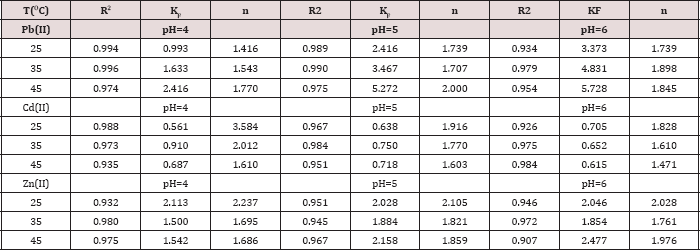The trend in qm values in poly(1,4-cyclohexanedimethylen phthalate) at pH= 6.00 and 25.0 oC is Pb(II) > Cd(II), in (mg/g) were 53.5>17.7 respectively . The trend in qm values for poly(1,4- cyclohexanedimethylene oxalate) at pH= 4.00 and 25.0 oC is Pb(II) > Cd(II) > Zn(II), in (mg/g) were 31.2 > 29.8 > 15.9, respectively . The trend for Pb(II) and Cd(II) ions in poly(hydroquinone oxalate), and in poly(neopentyl oxalate) polymer, were as follows: Pb(II)>Cd(II) [24-27] and thus the results similar in order the metal ion with our results in the literature  that is qm values 207.7 >30.7 >19.1 for Pb(II) >Zn(II) >Cd(II) in poly(bisphenol A oxalate). The results indicate that our polymer has reasonable qm values. In the Freundlich model, both KF and nare Freundlich constants, being indicative of the adsorption capacity and the adsorption intensity respectively. High value of n between (1.4-3.6) indicates that adsorption is good over the entire range of concentration studied, while small values of n means that the adsorption is good at high concentrations but much less at lower concentrations and the values of n were greater than one indicating that the adsorption was favorable. A greater value of KF indicates a higher capacity for the adsorption than smaller values .

The linear form for Dubinin-Radushkevich (D-R) isotherm has the following expression:R is the ideal gas constant (8.3145 J.mol-1.K-1) and T is the

absolute temperature (Kelvin). The values of p and q max are evaluated from the slope and intercept of the linear plot of lnq versus s2, where q max is related to the adsorption capacity and p is the constant related with the adsorption energy. The free energy of adsorption (E) is defined as the free energy change required for transferring one mole of ions from solution to the solid surface, this energy is calculated as follows:Table 5: Dubinnin-Radushkevich (D-R) isotherm parameters for Pb(II), Zn(II) and Cd(II).As illustrated in Tables 3-5, the values of E for Pb (II) are (0.1100.600 kJ/mol), for Cd(II) are (0.080 -0.200 kJ/mol) and for Zn(II) are (0.180-0.310kJ/mol). All values are less than 8.00kJ/mol, this indicates that physical forces affect adsorption. The result of the concentration variation isotherms of polymer (Table 5) and the plots of Dubinin-Radushkevich at pH= 4, 5 and 6 at 25 °C, 35 °C, 45 °C are shown in Figure 9.

The Effect of Temperature on The Uptake

The effect of varying temperature on the % uptake of metal ions was also investigated. The results are obtained by plotting % uptake at different pH against temperature and are presented in Figure 10. The results obtained showed that the adsorption process of Pb(II) , Zn(II) and Cd(II) onto the surface of the polymer is an endothermic process since the % uptake increases as the temperature increases nearly at all tested pH values.

Figure 9: Plots of Dubinnin-Radushkevich (D-R) isotherm for the adsorption of Pb(II) on the polymer at pH= 6.00 and a) T= 25 °C, b) T= 35oC, c) T=45 °C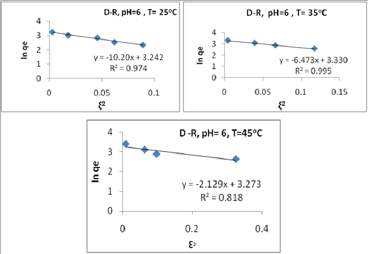Arrhenius Parameter

Table 6: The Arrhenius parameters for Pb(II), Zn(II) and Cd(II) at pH=6 and temperatures 25.0oC, 35.0oC, 45.0oC.Table 7: The Arrhenius parameters: Activation energy ( E) and pre-exponential factor (A) for Pb(II), Zn(II) and Cd(II) at pH=6 and temperatures 25oC, 35oC, 45oC.Arrhenius equation can be used to determine the activation energy and pre-exponential factor for a reaction were calculated from Figures 11 and shown in Table 6. The results A and Ea values that obtained in Figure 11 and Table 6 are calculated from Arrhenius equation:

Ln k = - Ea / R T + Ln A .....(4) (Table 7)

Where k is the rate constant, Ea activation energy, R gas constant and A pre-exponential factor. The values of A and Ea can be calculated from intercept and slope of a straight line of a plot of Ln k against 1/ T. The pre-exponential factor A is the constant of proportionality between the concentration of the reactants and the rate at which they collide. The activation energy Ea is the minimum kinetic energy required for a collision to result in reaction, through the more favorable molecular orientations. The factor exp (-Ea / RT) represents the fraction of molecular collisions that have an energy value equal to or greater than the activation energy Ea. At higher temperatures a larger portion of reactant molecules will have the required Ea to react. Thus, the reaction rates depend on Ea, the reactant orientations (relative positions) during collisions and the temperature. Both A and Ea are approximately constant over a moderate range of temperature (50K) . The order of Ea values for Pb, Zn and Cd is (19.2, 32.1, 83.9kJ/mol) respectively. This low value of Ea indicates a reaction rate slightly sensitive with temperature . So, we cannot explain the mechanism of the process from activation energy only but needed to use Eyring equation .

The Eyring equation (activated complex theory)

Determination of activation energy, entropy and enthalpy of activation by this equationThus, the above general form ofthe Eyring equation or activated Complex Theory equation, also known as Eyring-Polanyi equation in chemical kinetics, relates the reaction rate to temperature and is trivially equivalent to the empirical Arrhenius equation. The results were calculated from Figures 11 & 12 and shown in Table 8.

Figure 10: The temperature dependence of % uptake of Pb(II) , Zn(II) and Cd(II) ions at pH=6.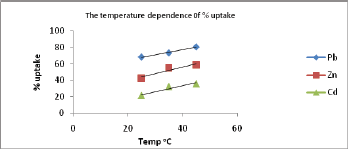Figure 11: The Arrhenius Plots of (a) Pb(II), (b) Zn(II) and (c) Cd(II) at pH= 6.00.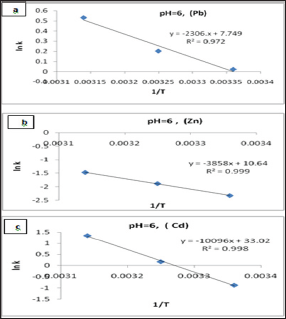Figure 12: The Activated Complex theory Plots of (a) Pb(II), (b) Zn(II) and (c) Cd (II) at pH= 6.00 .Table 8: Calculated thermodynamic parameters of activation (AH, ASt, AGt) at pH= 6 and 25oC.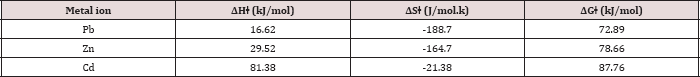The Eyring-Polanyi equation has been applied to rate processes and the calculation of values of enthalpies and entropies of activation without pointing out the significance of the obtained values and the difference between the activation energy values found by using the Arrhenius equation.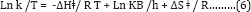A plot of (Ln k/T) versus 1/T gives a straight line with a slope of -ΔHǂ/R from which the enthalpy of activation can be derived and with intercept of ln (kB/h) +ΔSǂ/R from which the entropy of activation is derived. From the values of the free energy of activation the real energy requirements are known, thus, suggesting the use of the Eyring-Polanyi equation mainly as a tool for gaining a deeper understanding of the actual processes at work and not only as a tool for predicting reaction rates based on measured rate constants. This relation is usually used for the suggestion of a mechanism for a certain reaction in the following way: the certain reaction is performed at various temperatures where the reaction rate constant is measured. The pre-exponential factor A of Arrhenius equation has been related to ΔSǂ of Eyring equation.

Low values of lnA correspond to negative values of ΔSǂ, the activated complex in the transition state has a more organized, more ordered and more rigid structure than the reactants. This happens when bonds are formed or substances are absorbed, and high values of lnA correspond to positive (or less negative) values of ΔSǂ, a positive value for the entropy of activation indicates that the transition state is disordered (less organized), compared to the state of the reactants. This happens when bonds are broken or substances are desorbed. The calculated value of the entropy of activation is used for the suggestion of a mechanism i.e. in replacement reactions: Associative (ΔSǂ< 0), Dissociative (ΔSǂ> 0), Interchange (ΔSǂ = 0).  In our results in Table 8, the values of ΔSǂ for Pb(II), Zn(II) and Cd(II) are -188.7, -164.7, -21.3 J/mol.K, respectively all the values of ΔSǂ< 0 indicated that the process has Associative mechanism. The relation between Ea of the Arrhenius and ΔHǂ of the Eyring equation (activated complex theory) is:There was no comparison between Ea values and the ΔGǂ values that lead to the conclusion that Ea does not represent the full energetics of a process. Thus ΔGǂis the critical factor and not Ea. Thus, near room temperature (the thermodynamic temperature, 25 oC), Ea is roughly 2.5 kJ mol-1 larger than ΔHǂ.  Through Table (4.68) can be calculated divide between ΔHǂ and Ea for Pb(II), Zn(II) and Cd(II) that equal (2.6, 2.6, 2.5 kJ mol-1) respectively, there results indicated of the temperature independence on reaction rate and the temperature influences is room temperature 25 oC . For, so, we needed calculate the free energies of activation ΔGǂ. It has been found that ΔGǂ gives a more realistic/true value of the "activation the processes need in order to take place and not Ea or ΔSǂ alone. The free energy of activation ΔGǂ includes not only the ΔHǂ component (= Ea-RT) but also the ΔSǂ component that may be important. The term -TΔSǂ that hΔSǂo be added to ΔHǂ in order to give ΔGǂ which may be critical,

ΔGǂ = ΔHǂ- T ΔSǂ......(8)

Determines the spontaneity of the reaction ΔGǂ

ΔGǂ greater than zero reaction is spontaneous

ΔGǂ0 = system at equilibrium, no net change occurs

ΔGǂ less than zero reaction is not spontaneous . The results of ΔGǂ in Table 8 were explaining that all the values of ΔGǂ > 0. So, this process is spontaneous (physical process).

Distribution Coefficient (Kd)

The distribution coefficient is defined ΔSǂhe final concentration of metal ion in the sorbed form on polymer divided by its final concentration in solution. It is regarded a standard parameter in the assessment of the physicochemical behavior of metal ions between solid and liquid phases. It is calculated by the following equation.

Kd = qe / Ce = KL qm-KL qe........(9)

Table 9: Distribution coefficient for Pb(II) for different pH values and temperatures.Where Kd is the distribution coefficient (L/g). Thus, a plot of (qe / Ce) against (qe) should be a straight line with slope= - K and an intercept= qm K if Langmuir equation is applicable The distribution coefficients (Kd) is calculated for the polymer at different pH values (4.0, 5.0, and 6.0) and temperatures (25°C, 35°C, and 45°C) are given in Table 9.

Thermodynamics of Adsorption on the Polymer

In order to understand the possible adsorption mechanism involved in the removal process, thermodynamic functions for the system, including changes in Gibbs free energy (ΔG°), change in enthalpy of adsorption (ΔHǂ) and changes in entropy of adsorption (ΔS°), were calculated using the following equation Using the following equation: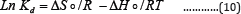Where Kd is the equilibrium constant, R is the gas constant and T is the temperature in Kelvin.

The plot of lnKd against 1/T for each metal ion gives a linear relationship, where the values of enthalpy (ΔHo) were entropy (ΔSo) are obtained from the slope and intercept of lnKd vs. 1/T plots. AGo was calculated at each temperature using the following equation:Table 9 present the data of distribution coefficients at different temperatures and pH values, while Figures 13 show the plots of lnKd versus 1/T (Table 10).

Figure 13: Plots of ln KdVs 1/T for Pb(II),(a) pH =4.00 (b) pH=5.00 and (c) pH=6.00.Table 10: Thermodynamic Functions for Pb(II) at T= 298.15 (K).The results of the studies on the influence of temperature on metal ions adsorption are presented in Table 10 above. The positive values of enthalpy indicate that the adsorption removal increased with increase of the solution temperature. This shows that the adsorption process is an endothermic one. AG°, ΔHǂ, and ΔS° are the thermodynamic functions related to the experiment conditions. Spontaneity and favorability of the adsorption process is established by decrease in Gibbs free energy values, AG°. The value of AG° between (0.16 - 1.04) indicates the degree of favorability of the adsorption process, so the values of AG presented in Table 10 indicate that the adsorption of Pb(II), Zn(II) and Cd(II) is a favorable process . All the values of AG° are very small and positive which suggests that the adsorption of metal ions onto polymer require some small amount of energy to convert reactants into products .

This is agreeing with values of Table 10 which represent to the degree of favorability of adsorption, the decrease of AG° values of Pb(II) >Zn(II) >Cd(II). As shown in Table 10 all AH values are positive this suggests the endothermic nature of metal adsorptions. One possible explanation of this is the well-known fact that heavy metal ions used are well solvated in water. In order for these ions to be adsorbed, they are denuded to some extent of the hydration sheath. This dehydration process of ions requires energy for removal of water from ions is essentially an endothermic process . We assume that the energy of dehydration exceeds the exothermicity of the ions attaching to the surface . The implicit assumption here is that after adsorption the environment of the metal ions is less aqueous than it was in the solution state.

The endothermic interactions between polymer surface and metal ions were accompanied by small positive values of entropy, which wΔS⫲he driving force for adsorption. The positive values of AS signify an increased state of randomness at the solid- solution interface following adsorption. Also the positive entropy of adsorption reflects the affinity of adsorbent for metal ions used. The adsorbed water molecules, which are displaced by the adsorbate species, gain more translational energy than is lost by the adsorbate ions, thus allowing the prevalence of randomness in the system . The entropy changes were most likely to be due to structural changes and adjustments in the adsorbate as well the adsorbent. The structural changes arise from the release of ions like H+ from the polymer surface into the solution and also from partial solvation of the metal ions in water. The adsorptions of Pb(II), Cd(II) and Zn(II) on polymer were associated with entropy decrease in conformity with the general situation of ions existing in a more chaotic random distribution in aqueous solution compared to their adsorbed and immobilized states .

Column Experiments

Metal ion uptake by the polymer

The metal ion uptake by the polymer using column experiment for Pb(II), Zn(II) and Cd(II) was determined at pH 6.0 and 25.0 oC, initial concentration of 150.0 mg/L and a flow rate 1 mL/4min. The uptake for metal ions is represented in Table 11.

Table 11: Metal iron uptake using column experiments.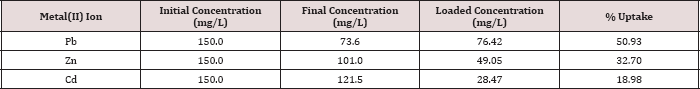It can be seen that the uptake capacities of the polymer with the metal ions fall in the order;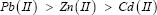This result is similar to the order of the metal ions in the batch experiment. However, the values of percent uptake for the metal ions in column experiment are lower than those obtained in batch experiments, because in order to achieve the complete saturation a much greater time is needed. On the other hand, there is no mechanical shaking associated with the column experiments, which result a decrease in percent of metal uptake.

Desorption Studies

Two eluting agents, 0.10 M HNO3 and 0.10 M EDTA were used for removal of metal ions, keeping the flow rate of elution 1 mL/4 min. The fluent was collected in five portions, 10.0mL for each portion; the results are expressed as percent recovery and represented in Table 12. The eluting agents react in two different ways: HNO3 act as proton-exchange agent and the second a complex-forming agent as EDTA. Depending on the values of the % accumulative recovery, in Table 12, the following trend was observed for the eluting agents of metal ions from the polymer:

0.1M HNO3 > 0.1M EDTA

Table 12: Desorption of Pb(II), Zn(II) and Cd(II) ions from the polymer.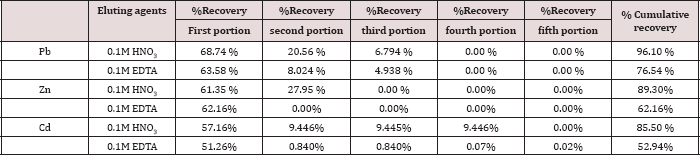The experiments confirmed that maximum metal desorption can be achieved with mineral acids in concentrations of 0.1M solutions. This could be attributed to cation exchange between the proton and metal sorbed. However, this method is more complex than protonation [8,38].

Conclusion

In this study, we prepared a polymer containing phthalate function group and capable of adsorbing the metal ion by solution polycondensation and characterization of poly(1,4- benzenedimethelene Phthalate). The structure and properties of polymer was confirmed by FT-IR, 1H NMR, 13C NMR, elemental analysis, SEM and thermal analysis. The sorption properties of the synthesized polymer toward Pb(II), Zn(II), and Cd(II) in aqueous solutions were examined under various experimental conditions using both batch and column experiments. The effective desorption for the metal ions was studied, and the coefficient of recovery of sorption ability was also investigated. The polymer has high sorption rate for Pb(II) observed during the first 24h with high percentage of uptakes toward Pb(II), Zn(II) and low percentage of uptakes toward Cd(II) ions. The influence of different pH on metals uptake showed that the metal-ion uptake by the polymer increased with increasing pH and reached a maximum at pH=6 for Pb(II), Zn(II), and Cd(II). The best conditions for adsorption of metal ions and maximum adsorption capacity (qm) on polymer surface are pH=6, T= 45°C and initial metal concentration of 150 ppm.

The obtained adsorption data showed fitting for Langmuir, Freundlich and Dubinin-Radushkevich adsorption isotherm models. The application of the Eyring equation to literature data i.e. the calculation of AH+, AS+ and AG+, has pointed out that in geochemical transformations it is necessary to calculate the entropy of activation along with the enthalpy of activation in order to fully characterize a process energetically. A column packed with the polymer has good metal uptake properties toward all metal ions, and followed the order: Pb(II) > Zn(II)> Cd(II) at pH 4.0 and 25 oC and flow rate 1 mL/4min. The efficiency of recovery of metal ions after adsorption can be carried out by treatment of the loaded polymer with 0.1M HNO3 and 0.1M EDTA with good percent recovery.

Acknowledgements

The authors would like to thank the Deanship of Academic Research and Quality Control (DAR) of The University of Jordan for supporting this work and all supervisors who worked in the improvement of this work.

CloseOnline Submission System

Submit ManuscriptClick here for Manuscript Sample TemplatesDrag and drop files here

or

( For multiple files submission, zip them in a single file to submit. For file zipping software Download )